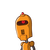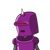# A 1.5 m tall boy is standing at some distance from a 30 m tall building. The angle of elevation from his eyes to the top of the b

A 1.5 m tall boy is standing at some distance from a 30 m tall building. The angle of elevation from his eyes to the top of the building increases from 30° to 60° as he walks towards the building. Find the distance he walked towards the building.​

### 2 thoughts on “A 1.5 m tall boy is standing at some distance from a 30 m tall building. The angle of elevation from his eyes to the top of the b”

1.tan 30=30-1.5/d

1/√3=28.5/d

d=28.5•√3

as equation 1

tan60=√3=28.5/d-x

√3(28.5*√3-x) =28.5

28.5*3-√3x=28.5

√3x=3*28.5-28.5

√3x=2*28.5=57

therefore x=57/√3=19*3/√3=19√3

19*1.732=32.908m

2.